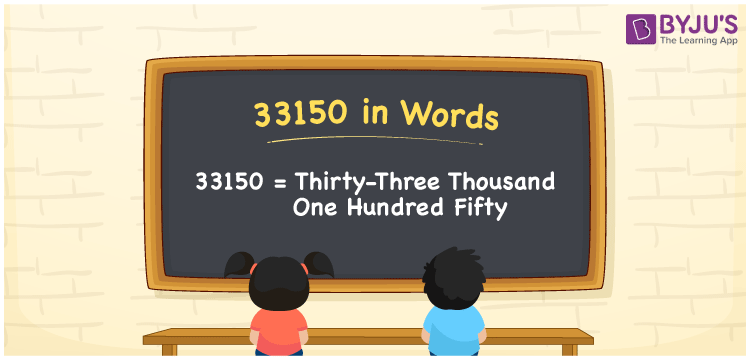# 33150 in Words

33150 in words can be written as Thirty-Three Thousand One Hundred Fifty. Using the English alphabet, students can convert the numbers into words. If you pay Rs. 33150 on a trip, then you can say that “I paid Thirty-Three Thousand One Hundred Fifty Rupees on a trip”. Numbers in words contain systematic representation to improve the analytical thinking abilities among students. 33150 can be read as “Thirty-Three Thousand One Hundred Fifty” in English.

 33150 in words Thirty-Three Thousand One Hundred Fifty Thirty-Three Thousand One Hundred Fifty in Numbers 33150

## 33150 in English Words## How to Write 33150 in Words?

Prepare a place value chart using the table below and convert numbers into words which is essential in our daily life activities.

 Ten Thousands Thousands Hundreds Tens Ones 3 3 1 5 0

33150 in expanded form can be written as:

3 x Ten Thousand + 3 x Thousand + 1 × Hundred + 5 × Ten + 0 × One

= 3 x 10000 + 3 x 1000 + 1 x 100 + 5 x 10 + 0 x 1

= 30000 + 3000 + 100 + 50

= 33150

= Thirty-Three Thousand One Hundred Fifty

Hence, 33150 in words is written as Thirty-Three Thousand One Hundred Fifty.

33150 is a natural number that precedes 33151 and succeeds 33149.

33150 in words – Thirty-Three Thousand One Hundred Fifty

Is 33150 an odd number? – No

Is 33150 an even number? – Yes

Is 33150 a perfect square number? – No

Is 33150 a perfect cube number? – No

Is 33150 a prime number? – No

Is 33150 a composite number? – Yes

## Frequently Asked Questions on 33150 in Words

Q1

### How to write 33150 in words?

33150 can be written in words as “Thirty-Three Thousand One Hundred Fifty”.
Q2

### Write Thirty-Three Thousand One Hundred Fifty in numbers.

Thirty-Three Thousand One Hundred Fifty in numbers can be written as 33150.
Q3

### Is 33150 an even number?

33150 is an even number as it is completely divisible by 2.